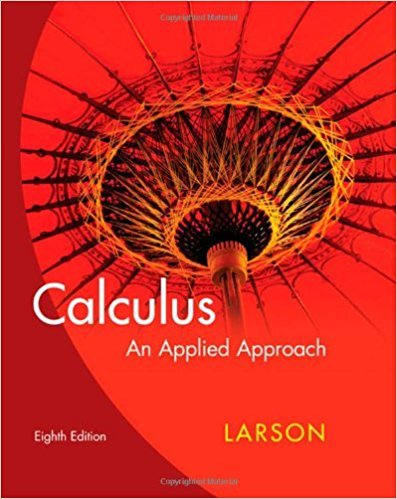×

×

Textbooks / Calculus / Calculus: An Applied Approach 8

# Calculus: An Applied Approach 8th Edition - Solutions by Chapter## Full solutions for Calculus: An Applied Approach | 8th Edition

ISBN: 9780618958252Calculus: An Applied Approach | 8th Edition - Solutions by Chapter

Solutions by Chapter
4 5 0 416 Reviews
##### ISBN: 9780618958252

The full step-by-step solution to problem in Calculus: An Applied Approach were answered by , our top Calculus solution expert on 03/05/18, 07:06PM. Calculus: An Applied Approach was written by and is associated to the ISBN: 9780618958252. This textbook survival guide was created for the textbook: Calculus: An Applied Approach , edition: 8. Since problems from 78 chapters in Calculus: An Applied Approach have been answered, more than 83117 students have viewed full step-by-step answer. This expansive textbook survival guide covers the following chapters: 78.

Key Calculus Terms and definitions covered in this textbook
• Absolute value of a complex number

The absolute value of the complex number z = a + b is given by ?a2+b2; also, the length of the segment from the origin to z in the complex plane.

• Degree of a polynomial (function)

The largest exponent on the variable in any of the terms of the polynomial (function)

• Fitting a line or curve to data

Finding a line or curve that comes close to passing through all the points in a scatter plot.

• Frequency

Reciprocal of the period of a sinusoid.

• Frequency table (in statistics)

A table showing frequencies.

• Intercept

Point where a curve crosses the x-, y-, or z-axis in a graph.

• Minor axis

The perpendicular bisector of the major axis of an ellipse with endpoints on the ellipse.

• Ordinary annuity

An annuity in which deposits are made at the same time interest is posted.

• Parametric equations

Equations of the form x = ƒ(t) and y = g(t) for all t in an interval I. The variable t is the parameter and I is the parameter interval.

• Product rule of logarithms

ogb 1RS2 = logb R + logb S, R > 0, S > 0,

A function that can be written in the form ƒ(x) = ax 2 + bx + c, where a, b, and c are real numbers, and a ? 0.

• Reflection through the origin

x, y and (-x,-y) are reflections of each other through the origin.

• Shrink of factor c

A transformation of a graph obtained by multiplying all the x-coordinates (horizontal shrink) by the constant 1/c or all of the y-coordinates (vertical shrink) by the constant c, 0 < c < 1.

• Square matrix

A matrix whose number of rows equals the number of columns.

• Symmetric matrix

A matrix A = [aij] with the property aij = aji for all i and j

• Transformation

A function that maps real numbers to real numbers.

• Trigonometric form of a complex number

r(cos ? + i sin ?)

• Work

The product of a force applied to an object over a given distance W = ƒFƒ ƒAB!ƒ.

• Ymin

The y-value of the bottom of the viewing window.

• Yscl

The scale of the tick marks on the y-axis in a viewing window.